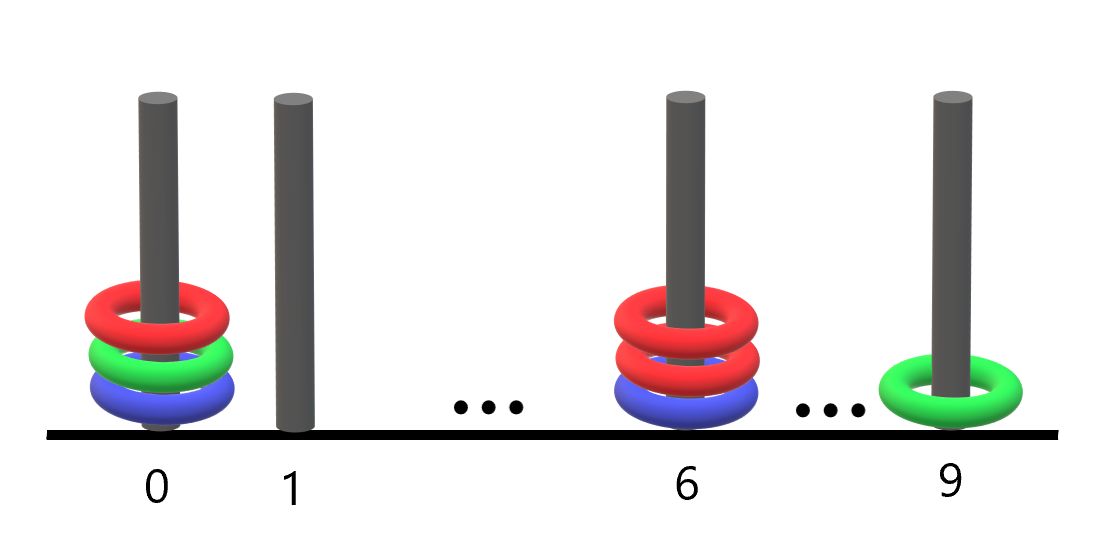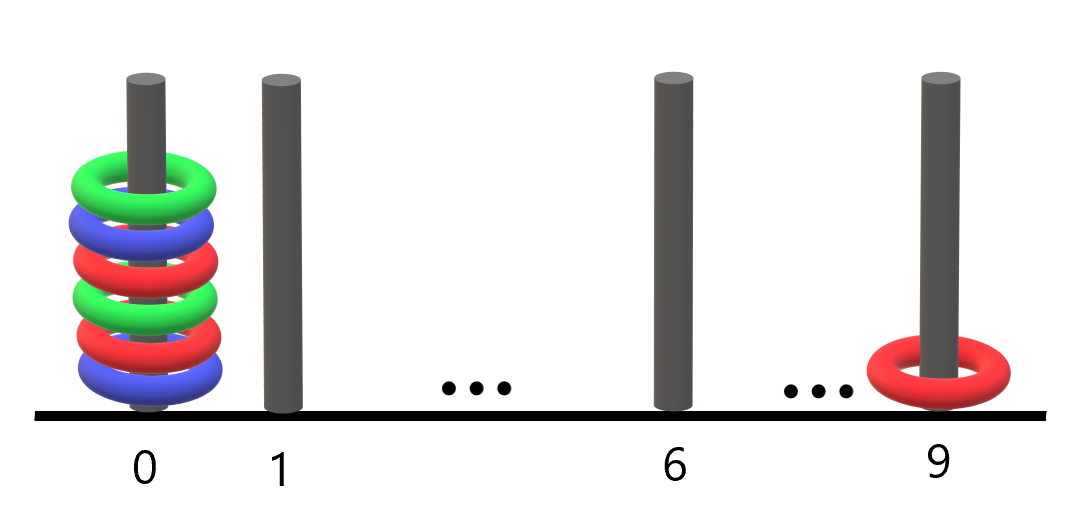2103. Rings and Rods

#### QUESTION:

There are n rings and each ring is either red, green, or blue. The rings are distributed across ten rods labeled from 0 to 9.

You are given a string rings of length 2n that describes the n rings that are placed onto the rods. Every two characters in rings forms a color-position pair that is used to describe each ring where:

The first character of the ith pair denotes the ith ring’s color (‘R’, ‘G’, ‘B’). The second character of the ith pair denotes the rod that the ith ring is placed on (‘0’ to ‘9’). For example, “R3G2B1” describes n == 3 rings: a red ring placed onto the rod labeled 3, a green ring placed onto the rod labeled 2, and a blue ring placed onto the rod labeled 1.

Return the number of rods that have all three colors of rings on them.

Example 1:``````Input: rings = "B0B6G0R6R0R6G9"
Output: 1
Explanation:
- The rod labeled 0 holds 3 rings with all colors: red, green, and blue.
- The rod labeled 6 holds 3 rings, but it only has red and blue.
- The rod labeled 9 holds only a green ring.
Thus, the number of rods with all three colors is 1.
``````

Example 2:``````Input: rings = "B0R0G0R9R0B0G0"
Output: 1
Explanation:
- The rod labeled 0 holds 6 rings with all colors: red, green, and blue.
- The rod labeled 9 holds only a red ring.
Thus, the number of rods with all three colors is 1.
``````

Example 3:

``````Input: rings = "G4"
Output: 0
Explanation:
Only one ring is given. Thus, no rods have all three colors.
``````

Constraints:

``````rings.length == 2 * n
1 <= n <= 100
rings[i] where i is even is either 'R', 'G', or 'B' (0-indexed).
rings[i] where i is odd is a digit from '0' to '9' (0-indexed).
``````

#### SOLUTION:

``````class Solution {
func countPoints(_ rings: String) -> Int {
var ringsArray = Array(rings)
var roadsArray:[Int] = Array(repeating: 0, count: 10)
var r:Int = 0b1
var g:Int = 0b10
var b:Int = 0b100
for index in stride(from: 0, to: ringsArray.count-1, by: 2) {
var light = 0
switch ringsArray[index] {
case "R":
light = r
case "G":
light = g
case "B":
light = b
default:
light = 0
}